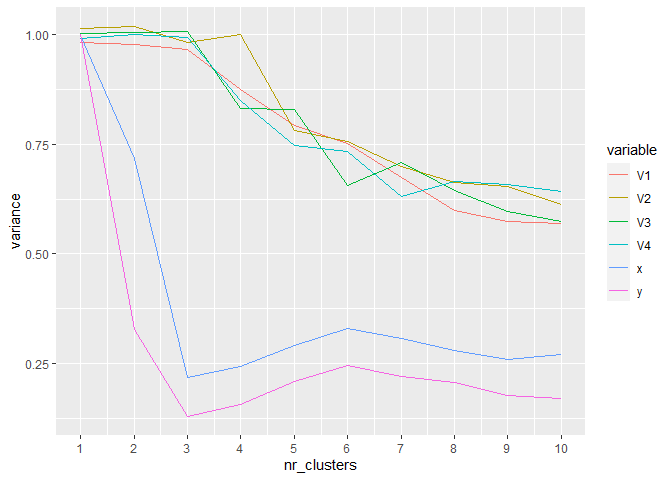# Simulated data with missings

We start by loading R packages used in this example

required_packages <- Hmisc::Cs(psych,ggplot2,ggExtra,tidyr,Hmisc,tictoc,ClusterR,copula,dplyr,corrplot,ClustImpute)
lapply(required_packages, require, character.only = TRUE)
#> []
#>  TRUE
#>
#> []
#>  TRUE
#>
#> []
#>  TRUE
#>
#> []
#>  TRUE
#>
#> []
#>  TRUE
#>
#> []
#>  TRUE
#>
#> []
#>  TRUE
#>
#> []
#>  TRUE
#>
#> []
#>  TRUE
#>
#> []
#>  TRUE
#>
#> []
#>  TRUE

First we create a random dataset with some structure and a few uncorrelated variables

### Random Dataset
set.seed(739)
n <- 7500 # numer of points
nr_other_vars <- 4
mat <- matrix(rnorm(nr_other_vars*n),n,nr_other_vars)
me<-4 # mean
x <- c(rnorm(n/3,me/2,1),rnorm(2*n/3,-me/2,1))
y <- c(rnorm(n/3,0,1),rnorm(n/3,me,1),rnorm(n/3,-me,1))
true_clust <- c(rep(1,n/3),rep(2,n/3),rep(3,n/3)) # true clusters
dat <- cbind(mat,x,y)
dat<- as.data.frame(scale(dat)) # scaling
summary(dat)
#>        V1                 V2                  V3                 V4
#>  Min.   :-3.40352   Min.   :-4.273673   Min.   :-3.82710   Min.   :-3.652267
#>  1st Qu.:-0.67607   1st Qu.:-0.670061   1st Qu.:-0.66962   1st Qu.:-0.684359
#>  Median : 0.01295   Median :-0.006559   Median :-0.01179   Median : 0.001737
#>  Mean   : 0.00000   Mean   : 0.000000   Mean   : 0.00000   Mean   : 0.000000
#>  3rd Qu.: 0.67798   3rd Qu.: 0.684672   3rd Qu.: 0.67221   3rd Qu.: 0.687404
#>  Max.   : 3.35535   Max.   : 3.423416   Max.   : 3.80557   Max.   : 3.621530
#>        x                 y
#>  Min.   :-2.1994   Min.   :-2.151001
#>  1st Qu.:-0.7738   1st Qu.:-0.975136
#>  Median :-0.2901   Median : 0.009932
#>  Mean   : 0.0000   Mean   : 0.000000
#>  3rd Qu.: 0.9420   3rd Qu.: 0.975788
#>  Max.   : 2.8954   Max.   : 2.265420

One can clearly see the three clusters of the randomly generated data:

dat4plot <- dat
dat4plot$true_clust_fct <- factor(true_clust) p_base <- ggplot(dat4plot,aes(x=x,y=y,color=true_clust_fct)) + geom_point() ggExtra::ggMarginal(p_base, groupColour = TRUE, groupFill = TRUE)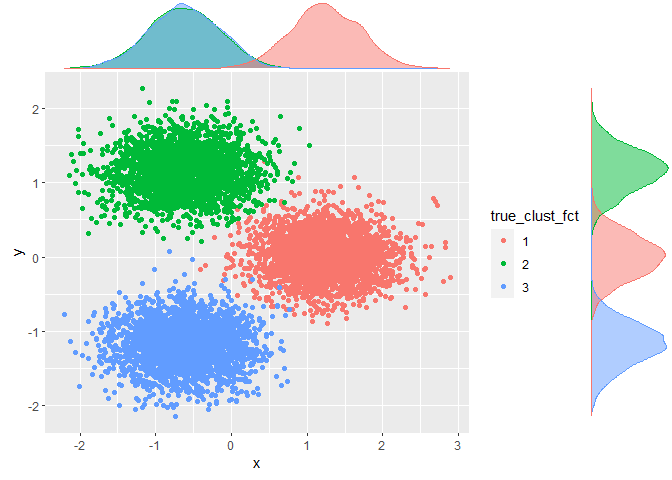We create a 20% missings using a custom function dat_with_miss <- miss_sim(dat,p=.2,seed_nr=120) summary(dat_with_miss) #> V1 V2 V3 V4 #> Min. :-3.4035 Min. :-4.2737 Min. :-3.8271 Min. :-3.5844 #> 1st Qu.:-0.6756 1st Qu.:-0.6757 1st Qu.:-0.6634 1st Qu.:-0.6742 #> Median : 0.0163 Median :-0.0104 Median :-0.0092 Median : 0.0194 #> Mean : 0.0024 Mean :-0.0063 Mean : 0.0027 Mean : 0.0117 #> 3rd Qu.: 0.6886 3rd Qu.: 0.6683 3rd Qu.: 0.6774 3rd Qu.: 0.7010 #> Max. : 3.2431 Max. : 3.4234 Max. : 3.8056 Max. : 3.6215 #> NA's :1513 NA's :1499 NA's :1470 NA's :1486 #> x y #> Min. :-2.1994 Min. :-2.1510 #> 1st Qu.:-0.7636 1st Qu.:-0.9745 #> Median :-0.2955 Median : 0.0065 #> Mean : 0.0022 Mean :-0.0019 #> 3rd Qu.: 0.9473 3rd Qu.: 0.9689 #> Max. : 2.8954 Max. : 2.2654 #> NA's :1580 NA's :1516 mis_ind <- is.na(dat_with_miss) # missing indicator The correlation matrix of the missing indicator shows that the missings are correlated - thus we are not in a missing completely at random stetting: corrplot::corrplot(cor(mis_ind),method="number")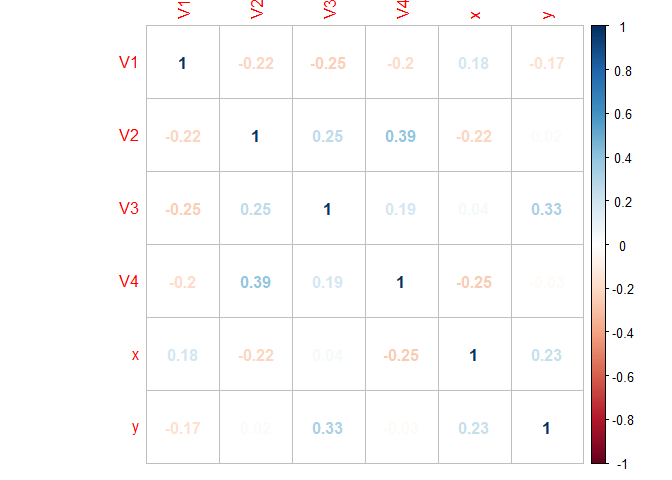# Median or random imputation Clearly, an imputation with the median value does a pretty bad job here. All imputed values lie on either of the two axes thereby completely distorting the marginal distributions: dat_median_imp <- dat_with_miss for (j in 1:dim(dat)) { dat_median_imp[,j] <- Hmisc::impute(dat_median_imp[,j],fun=median) } imp <- factor(pmax(mis_ind[,5],mis_ind[,6]),labels=c("Original","Imputed")) # point is imputed if x or y is imputed p_median_imp <- ggplot(dat_median_imp) + geom_point(aes(x=x,y=y,color=imp)) ggExtra::ggMarginal(p_median_imp,groupColour = TRUE, groupFill = TRUE) #> Don't know how to automatically pick scale for object of type impute. Defaulting to continuous. #> Don't know how to automatically pick scale for object of type impute. Defaulting to continuous. #> Don't know how to automatically pick scale for object of type impute. Defaulting to continuous. #> Don't know how to automatically pick scale for object of type impute. Defaulting to continuous.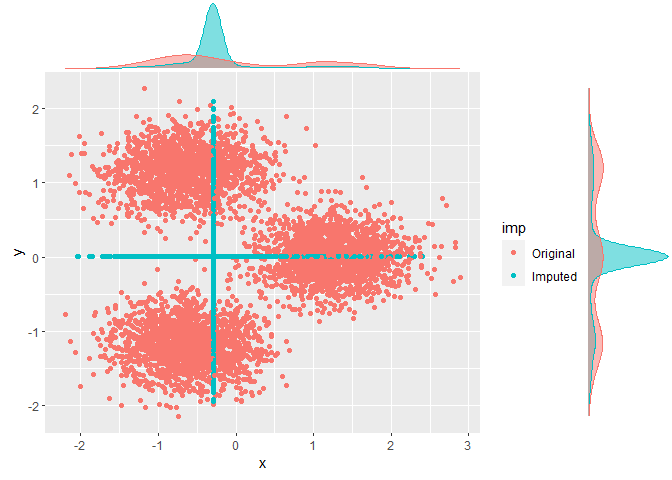But also a random imputation is not much better: it creates plenty of points in areas with no data. Note how a simple check of the marginal distributions would not reveal this issue! dat_random_imp <- dat_with_miss for (j in 1:dim(dat)) { dat_random_imp[,j] <- impute(dat_random_imp[,j],fun="random") } imp <- factor(pmax(mis_ind[,5],mis_ind[,6]),labels=c("Original","Imputed")) # point is imputed if x or y is imputed p_random_imp <- ggplot(dat_random_imp) + geom_point(aes(x=x,y=y,color=imp)) ggExtra::ggMarginal(p_random_imp,groupColour = TRUE, groupFill = TRUE) #> Don't know how to automatically pick scale for object of type impute. Defaulting to continuous. #> Don't know how to automatically pick scale for object of type impute. Defaulting to continuous. #> Don't know how to automatically pick scale for object of type impute. Defaulting to continuous. #> Don't know how to automatically pick scale for object of type impute. Defaulting to continuous.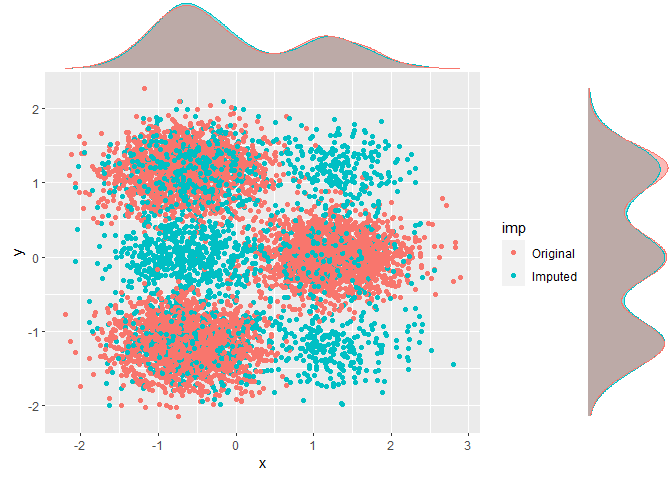A cluster base on random imputation will thus not provide good results (even if we “know” the number of clusters, which is 3 in this case). Note how the marginal distribution for y differs from the first chart of this vignette where we show the true clusters instead of the predicted clusters from a random imputation. tictoc::tic("Clustering based on random imputation") cl_compare <- KMeans_arma(data=dat_random_imp,clusters=3,n_iter=100,seed=751) tictoc::toc() #> Clustering based on random imputation: 0 sec elapsed dat_random_imp$pred <- predict_KMeans(dat_random_imp,cl_compare)
p_random_imp <- ggplot(dat_random_imp) + geom_point(aes(x=x,y=y,color=factor(pred)))
ggExtra::ggMarginal(p_random_imp,groupColour = TRUE, groupFill = TRUE)
#> Don't know how to automatically pick scale for object of type impute. Defaulting to continuous.
#> Don't know how to automatically pick scale for object of type impute. Defaulting to continuous.
#> Don't know how to automatically pick scale for object of type impute. Defaulting to continuous.
#> Don't know how to automatically pick scale for object of type impute. Defaulting to continuous.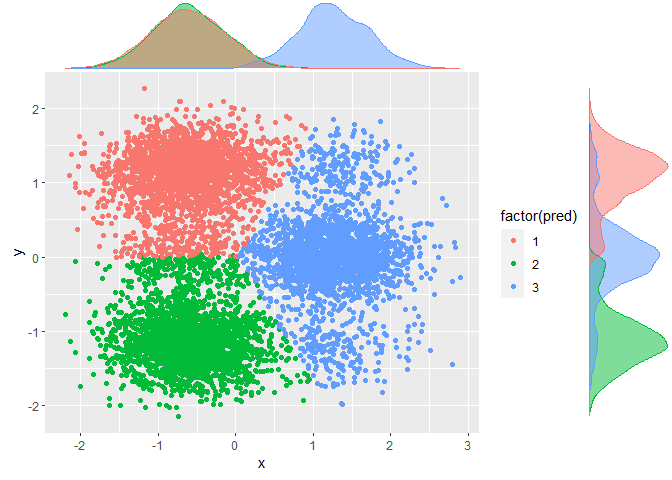# Solution: ClustImpute

We’ll now use ClustImpute and also measure the run-time. In short, the algorithm follows these steps

1. It replaces all NAs by random imputation, i.e., for each variable with missings, it draws from the marginal distribution of this variable not taking into account any correlations with other variables
2. Weights <1 are used to adjust the scale of an observation that was generated in step 1. The weights are calculated by a (linear) weight function that starts near zero and converges to 1 at n_end.
3. A k-means clustering is performed with a number of c_steps steps starting with a random initialization.
4. The values from step 2 are replaced by new draws conditionally on the assign cluster from step 3.
5. Steps 2-4 are repeated nr_iter times in total. The k-means clustering in step 3 uses the previous cluster centroids for initialization.
6. After the last draws a final k-means clustering is performed.

The intuition is that observation should be clustered with other observations mainly based on their observed values, while the resulting clusters provide donors for the missing value imputation, so that subsequently all variables can be used for the clustering.

nr_iter <- 10 # iterations of procedure
n_end <- 10 # step until convergence of weight function to 1
nr_cluster <- 3 # number of clusters
c_steps <- 50 # numer of cluster steps per iteration
tictoc::tic("Run ClustImpute")
res <- ClustImpute(dat_with_miss,nr_cluster=nr_cluster, nr_iter=nr_iter, c_steps=c_steps, n_end=n_end)
tictoc::toc()
#> Run ClustImpute: 0.32 sec elapsed

To get an overview of what ClustImpute you may run the following commands:

res
summary(res)
attributes(res)

We’ll first look at the complete data and clustering results. Quite obviously, it gives better results than median / random imputation.

p_clustimpute <- ggplot(res$complete_data,aes(x,y,color=factor(res$clusters))) + geom_point()
ggExtra::ggMarginal(p_clustimpute,groupColour = TRUE, groupFill = TRUE)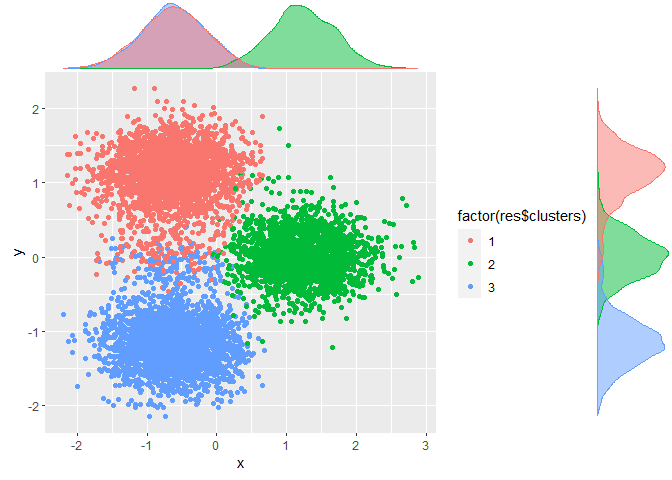## Marginal distributions by cluster and feature

In this toy example we already know that we only have to focus on the “real” features x and y, but this will not be the case in practice. Therefore one can also plot the distributions by cluster and feature.The orange bars are showing the cluster centroids. The plots for x and y are in line what we have seen above, and there is not much difference in the noise features V1 to V4 as one would expect.

plot(res)+xlim(-2.5,2.5)
#> Warning: Removed 385 rows containing non-finite values (stat_bin).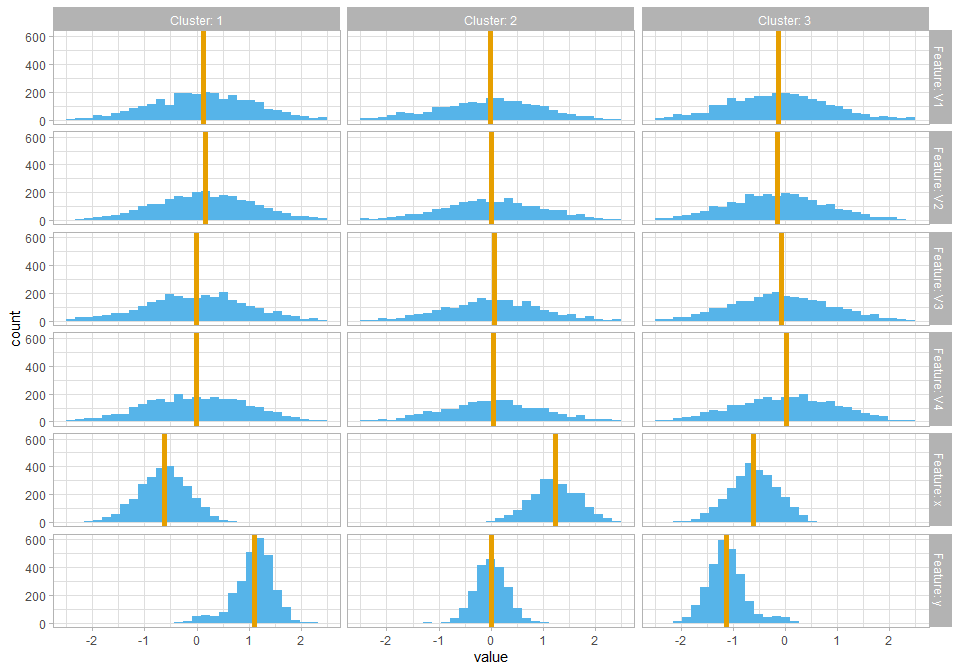Alternatively once can also visualize the marginal distributions with a box-plot.

plot(res, type="box")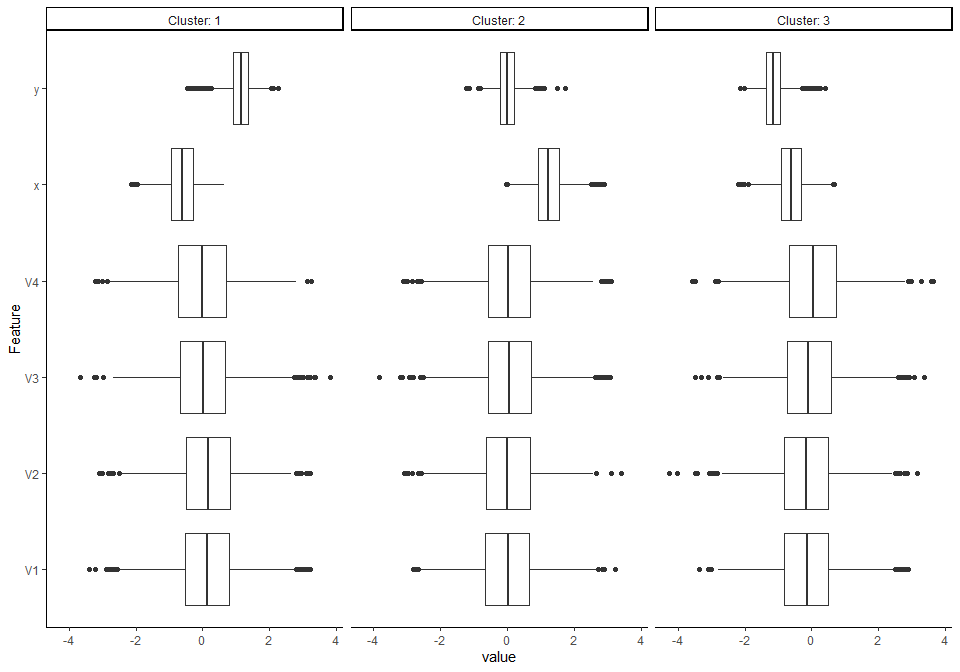## Traceplot of mean and variance

Packages like MICE compute a traceplot of mean and variance for various chain. Here we only have a single realization and thus re-run ClustImpute with various seeds to obtain different realizations.

res2 <- ClustImpute(dat_with_miss,nr_cluster=nr_cluster, nr_iter=nr_iter, c_steps=c_steps, n_end=n_end,seed_nr = 2)
res3 <- ClustImpute(dat_with_miss,nr_cluster=nr_cluster, nr_iter=nr_iter, c_steps=c_steps, n_end=n_end,seed_nr = 3)
mean_all <- rbind(res$imp_values_mean,res2$imp_values_mean,res3$imp_values_mean) sd_all <- rbind(res$imp_values_sd,res2$imp_values_sd,res3$imp_values_sd)
mean_all <- cbind(mean_all,seed=rep(c(150519,2,3),each=11))
sd_all <- cbind(sd_all,seed=rep(c(150519,2,3),each=11))

The realizations mix nicely with each other.

ggplot(as.data.frame(mean_all)) + geom_line(aes(x=iter,y=V1,color=factor(seed))) + ggtitle("Mean")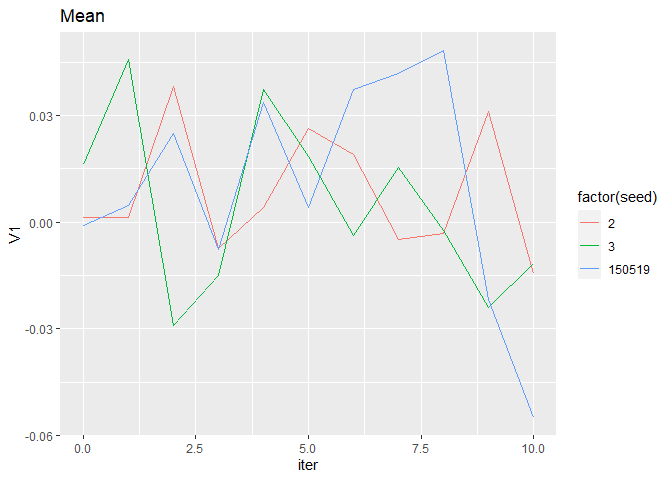ggplot(as.data.frame(sd_all)) + geom_line(aes(x=iter,y=V1,color=factor(seed))) + ggtitle("Std. dev.")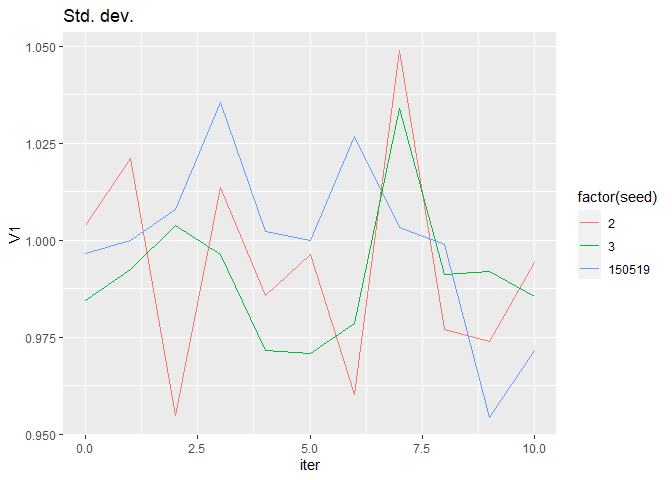# Quality of imputation and cluster results

## External validation: rand index

Below we compare the rand index between true and fitted cluster assignment. For all cases we obtain

external_validation(true_clust, res$clusters) #>  0.6210542 It is considerably lower for random imputation: class(dat_random_imp$pred) <- "numeric"
external_validation(true_clust, dat_random_imp$pred) #>  0.5908074 Of course, it is much higher on the (small number of) complete cases. ## complete cases idx <- which(complete.cases(dat_with_miss)==TRUE) sprintf("Number of complete cases is %s",length(idx)) #>  "Number of complete cases is 2181" sprintf("Rand index for this case %s", external_validation(true_clust[idx], res$clusters[idx]))
#>  "Rand index for this case 0.979412552022036"

The function also computes a variety of other stats

external_validation(true_clust, res$clusters,summary_stats = TRUE) #> #> ---------------------------------------- #> purity : 0.8597 #> entropy : 0.424 #> normalized mutual information : 0.5708 #> variation of information : 1.3557 #> normalized var. of information : 0.6006 #> ---------------------------------------- #> specificity : 0.8694 #> sensitivity : 0.7541 #> precision : 0.7426 #> recall : 0.7541 #> F-measure : 0.7483 #> ---------------------------------------- #> accuracy OR rand-index : 0.8309 #> adjusted-rand-index : 0.6211 #> jaccard-index : 0.5978 #> fowlkes-mallows-index : 0.7483 #> mirkin-metric : 9507830 #> ---------------------------------------- #>  0.6210542 ## Variance reduction We assess quality of clusters, we compute the sum of squares within each cluster, sum up this value and compare it by the total sum of squares. res_var <- var_reduction(res) res_var$Variance_reduction
#>  0.2737426
res_var$Variance_by_cluster #> # A tibble: 1 x 6 #> V1 V2 V3 V4 x y #> <dbl> <dbl> <dbl> <dbl> <dbl> <dbl> #> 1 0.967 0.982 1.01 0.993 0.218 0.130 We se a reduction of about 27% using only 3 clusters, most strikingly for x and y because that these variables define the subspace of the true clusters. More clusters will capture the random distribution of the other variables res <- ClustImpute(dat_with_miss,nr_cluster=10, nr_iter=nr_iter, c_steps=c_steps, n_end=n_end) res_var <- var_reduction(res) res_var$Variance_reduction
#>  0.5208357
res_var$Variance_by_cluster #> # A tibble: 1 x 6 #> V1 V2 V3 V4 x y #> <dbl> <dbl> <dbl> <dbl> <dbl> <dbl> #> 1 0.569 0.612 0.574 0.643 0.270 0.170 We’ll do this exercise for a several values of nr_cluster (and need a helper function for that since X is an argument of ClustImpute) ClustImpute2 <- function(dataFrame,nr_cluster, nr_iter=10, c_steps=1, wf=default_wf, n_end=10, seed_nr=150519) { return(ClustImpute(dataFrame,nr_cluster, nr_iter, c_steps, wf, n_end, seed_nr)) } res_list <- lapply(X=1:10,FUN=ClustImpute2,dataFrame=dat_with_miss, nr_iter=nr_iter, c_steps=c_steps, n_end=n_end) ..and put the variances by cluster in a table tmp <- var_reduction(res_list[]) var_by_clust <- tmp$Variance_by_cluster
for (k in 2:10) {
tmp <- var_reduction(res_list[[k]])
var_by_clust <- rbind(var_by_clust,tmp$Variance_by_cluster) } var_by_clust$nr_clusters <- 1:10

While there is a rather gradual improvement for the other variables, x and y have a minimum showing optimality for these variables. Such a plot clearly indicates that 3 clusters are a good choice for this data set.

data2plot <- tidyr::gather(var_by_clust,key = "variable", value = "variance", -dplyr::one_of("nr_clusters"))
ggplot(data2plot,aes(x=nr_clusters,y=variance,color=variable)) + geom_line() + scale_x_continuous(breaks=1:10)